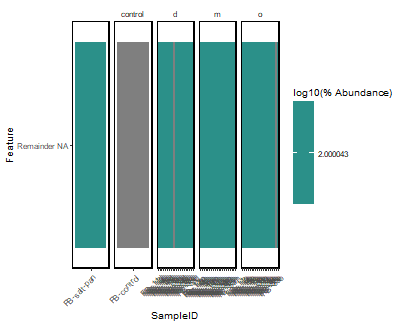# Qiime2R Heatmap construction challenges

I'm using Qiime2 2022.2. I have been trying to come up with a heat map in qiime2r following a tutorial by @jbisanz. Here is an output.
Please help to guide me through, where I could have missed it. Below is the code I used to run the analysis.

library(tidyverse)
library(qiime2R)

SVs<-apply(SVs, 2, function(x) x/sum(x)*100)

SVsToPlot<-
data.frame(MeanAbundance=rowMeans(SVs)) %>%
rownames_to_column("Feature.ID") %>%
arrange(desc(MeanAbundance)) %>%
top_n(30, MeanAbundance) %>%
pull(Feature.ID)

SVs %>%
as.data.frame() %>%
rownames_to_column("Feature.ID") %>%
gather(-Feature.ID, key="SampleID", value="Abundance") %>%
mutate(Feature.ID=if_else(Feature.ID %in% SVsToPlot, Feature.ID, "Remainder")) %>%
group_by(SampleID, Feature.ID) %>%
summarize(Abundance=sum(Abundance)) %>%
mutate(NormAbundance=log10(Abundance+0.01)) %>%
left_join(taxonomy) %>%
mutate(Feature=paste(Feature.ID, Taxon)) %>%
mutate(Feature=gsub("[kpcofgs]__", "", Feature)) %>%
ggplot(aes(x=SampleID, y=Feature, fill=NormAbundance)) +
geom_tile() +
facet_grid(~`depth`, scales="free_x") +
theme_q2r() +
theme(axis.text.x=element_text(angle=45, hjust=1)) +
scale_fill_viridis_c(name="log10(% Abundance)")
ggsave("heatmap.pdf", height=4, width=11, device="pdf")

Thank you in advance

This topic was automatically closed 31 days after the last reply. New replies are no longer allowed.